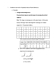# STATS 250 Lecture Notes - Lecture 4: Unimodality, French Fries, Standard Deviation

40 views1 pages
School
Department
Course
Professorazureswan549 and 37 others unlocked49
Verified Note
49 documents

## Document Summary

Bell-shaped somewhat symmetric unimodal representation of data. Standard deviation roughly the average distance the observations fall from the mean: actually the square root of the squared deviation of observations from the mean s = sample standard deviation = = S2 = variance: emphasize the standard deviation because it is in the original units. Ex: the weights of small orders of french fries are roughly 6. 1 g away from their mean weight of 73. 5g. S=0 means that there is no variability or spread; all observations have the same value. Like the mean, s is very sensitive to outliers or extreme observations. Whenever you use the mean as the measure of center, use standard deviation as a measure of spread. Use the mean and standard deviation for symmetric bell-shaped distributions. Five-number summary is better for skewed distributions or outliers. Statistic numerical summary based on a sample: sample mean, sample standard deviation.

## Get access

\$8 USD/m\$10 USD/m
Billed \$96 USD annuallyHomework Help
Study Guides
Textbook Solutions
Class Notes
Textbook Notes
Booster Class
Class+
\$8 USD/m
Billed \$96 USD annuallyHomework Help
Study Guides
Textbook Solutions
Class Notes
Textbook Notes
Booster Class